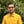Trusted answers to developer questions
Trusted Answers to Developer Questions

Related Tags

python
math
communitycreator

# What is math.atan() in Python?Harris Amjad

Grokking Modern System Design Interview for Engineers & Managers

Ace your System Design Interview and take your career to the next level. Learn to handle the design of applications like Netflix, Quora, Facebook, Uber, and many more in a 45-min interview. Learn the RESHADED framework for architecting web-scale applications by determining requirements, constraints, and assumptions before diving into a step-by-step design process.

The atan() functionalso called the arc tangent function returns the inverse tangent of a number. To be more specific, it returns the inverse tangent of a number in radians.

Figure 1 shows the mathematical representation of the atan() function and Figure 2 shows the visual representation of the atan() function.

Figure 1: Mathematical representation of inverse tangent function
Figure 2: Visual representation of inverse tangent function

Note: The math module is required for this function.

Tto convert radians to degrees, use:

degrees = radians * ( 180.0 / pi )


## Syntax

atan(num)


## Parameter

This function requires a number as a parameter. The parameter must be a double value between -1 and 1, i.e., -1 <= parameter <= 1.

## Return value

atan() will return the inverse tangent of a number (radians) that is sent as a parameter. The return values lies in interval [-pi/2,pi/2] radians.

## Example

import math#positive number in radiansprint "The value of atan(0.5) : ",  math.atan(0.5), "Radians"#negative number in radiansprint "The value of atan(-0.5) : ",  math.atan(-0.5), "Radians"#applying atan() and then converting the result in radians to degrees#radians = 1.0#PI = 3.14159265result = math.atan(1.0) * (180.0 / math.pi)print "The value of atan(1.0) : ", result ,"Degrees"

RELATED TAGS

python
math
communitycreator

CONTRIBUTORHarris Amjad

Grokking Modern System Design Interview for Engineers & Managers

Ace your System Design Interview and take your career to the next level. Learn to handle the design of applications like Netflix, Quora, Facebook, Uber, and many more in a 45-min interview. Learn the RESHADED framework for architecting web-scale applications by determining requirements, constraints, and assumptions before diving into a step-by-step design process.

Keep Exploring

Learn in-demand tech skills in half the time